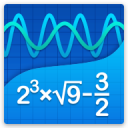# Mathlab Calc 2023.03.163 [paid]

### Description

Graphing calculator with algebra. Essential tool for school and college. Replaces bulky and expensive handheld graphing calculators.Multiple functions on a graph, polar graphs, graphing of implicit functions, values and slopes, roots, extremes, intersections. Algebra: polynomials, polynomial equation solving, matrices, fractions, derivatives, complex numbers and more. Shows results as you type. Use menu to switch between modes.Free version requires internet connection and contains ads! \$5.99 in-app upgrade to PRO. Contact us if you need multiple licenses for a school or organization.Help site with instructions and examples: http://help.mathlab.us
If you have a question, send email to [email protected] CALCULATOR
* arithmetic expressions +,-,*,/,÷
* square root, cube and higher roots (hold root key)
* exponent, logarithms (ln, log)
* trigonometric functions sin π/2, cos 30°, ...
* hyperbolic functions sinh, cosh, tanh, ... (hold "e" key to switch)
* inverse functions (hold direct function key)
* complex numbers, all functions support complex arguments
* derivatives sin x' = cos x, ... (hold x^n key)
* scientific notation (enable in menu)
* percent mode
* multiple functions graphing
* implicit functions up to 2nd degree (ellipse 2x^2+3y^2=1, etc.)
* polar graphs (r=cos2θ)
* parametric functions, enter each on new line (x=cos t, y=sin t)
* function roots and critical points on a graph, tap legend to turn on and off (top left corner), use menu to display as a list
* graph intersections (x^2=x+1)
* tracing function values and slopes
* scrollable and resizable graphs
* pinch to zoom
* fullscreen graphs in landscape orientation
* function tables
* save graphs as images
* save tables as csvFRACTION CALCULATOR
* simple and complex fractions 1/2 + 1/3 = 5/6
* mixed numbers, use space to enter values 3 1/2ALGEBRA CALCULATOR
* linear equations x+1=2 -> x=1
* quadratic equations x^2-1=0 -> x=-1,1
* approximate roots of higher polynomials
* systems of linear equations, write one equation per line, x1+x2=1, x1-x2=2
* polynomial long division
* polynomial expansion, factoringMATRIX CALCULATOR
* matrix and vector operations
* dot product (hold *), cross product
* determinant, inverse, norm, transpose, traceLIBRARY
* user defined constants and functions (PRO)
Free DownloadDownload by QR Code